Anzeige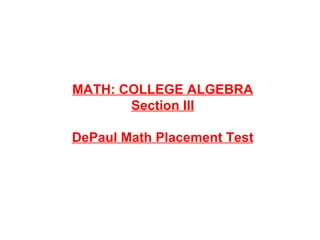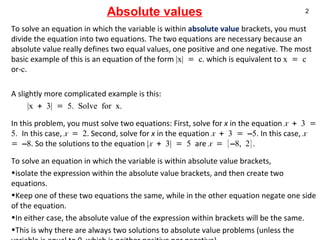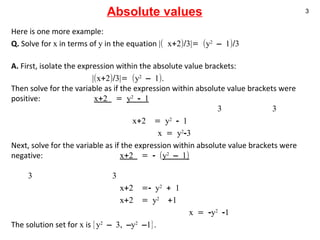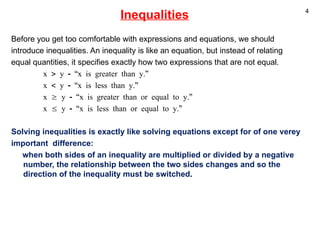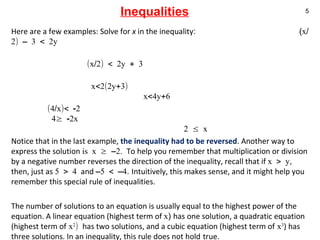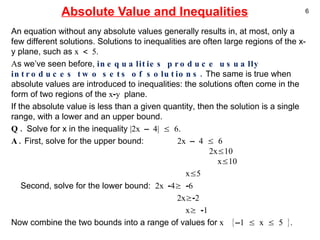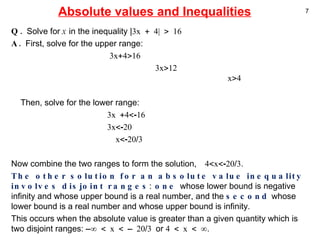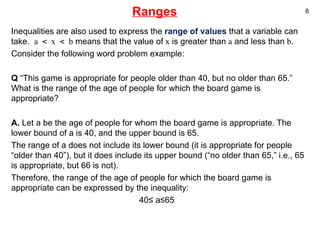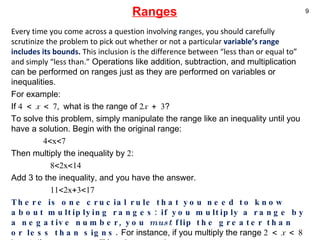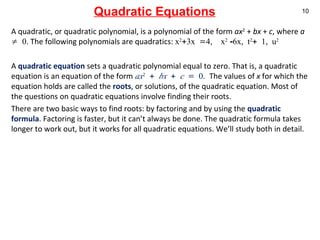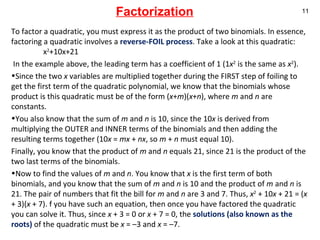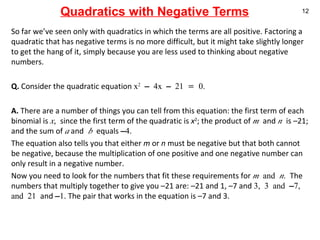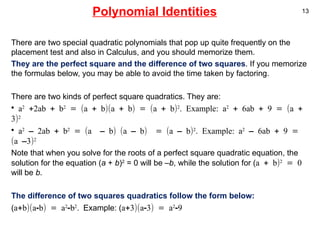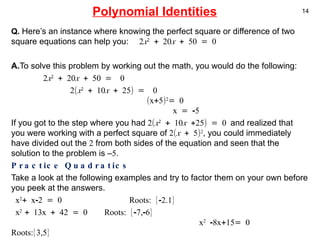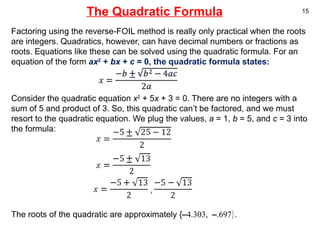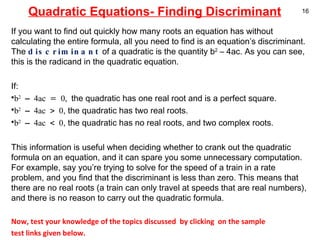1 von 16
Anzeige

### .

1. MATH: COLLEGE ALGEBRA Section III DePaul Math Placement Test
2. Absolute values Here is one more example: Q. Solve for x in terms of y in the equation |( x+2)/3|= (y 2 – 1)/3 A. First, isolate the expression within the absolute value brackets: |(x+2)/3|= (y 2 – 1). Then solve for the variable as if the expression within absolute value brackets were positive: x+2 = y 2 - 1 3 3 x+2 = y 2 - 1 x = y 2 -3 Next, solve for the variable as if the expression within absolute value brackets were negative: x+2 = - ( y 2 – 1) 3 3 x+2 =- y 2 + 1 x+2 = y 2 +1 x = -y 2 -1 The solution set for x is {y 2 – 3, –y 2 –1}.
3. Inequalities Before you get too comfortable with expressions and equations, we should introduce inequalities. An inequality is like an equation, but instead of relating equal quantities, it specifies exactly how two expressions that are not equal. x > y - “x is greater than y.” x < y - “x is less than y.” x ≥ y - “x is greater than or equal to y.” x ≤ y - “x is less than or equal to y.” Solving inequalities is exactly like solving equations except for of one verey important difference: when both sides of an inequality are multiplied or divided by a negative number, the relationship between the two sides changes and so the direction of the inequality must be switched .
4. Inequalities Here are a few examples: Solve for x in the inequality : ( x/2) – 3 < 2y (x/2) < 2y + 3 x<2(2y+3) x<4y+6 (4/x)< -2 4≥ -2x 2 ≤ x Notice that in the last example, the inequality had to be reversed . Another way to express the solution is x ≥ –2. To help you remember that multiplication or division by a negative number reverses the direction of the inequality, recall that if x > y , then, just as 5 > 4 and –5 < –4 . Intuitively, this makes sense, and it might help you remember this special rule of inequalities. The number of solutions to an equation is usually equal to the highest power of the equation. A linear equation (highest term of x ) has one solution, a quadratic equation (highest term of x 2 ) has two solutions, and a cubic equation (highest term of x 3 ) has three solutions. In an inequality, this rule does not hold true.
5. Absolute Value and Inequalities An equation without any absolute values generally results in, at most, only a few different solutions. Solutions to inequalities are often large regions of the x-y plane, such as x < 5. A s we’ve seen before, inequalities produce usually introduces two sets of solutions. The same is true when absolute values are introduced to inequalities: the solutions often come in the form of two regions of the x-y plane. If the absolute value is less than a given quantity, then the solution is a single range, with a lower and an upper bound. Q. Solve for x in the inequality |2x – 4| ≤ 6 . A. First, solve for the upper bound: 2x – 4 ≤ 6 2x≤10 x≤10 x≤5 Second, solve for the lower bound: 2x -4≥ -6 2x≥-2 x≥ -1 Now combine the two bounds into a range of values for x {–1 ≤ x ≤ 5 }.
6. Absolute values and Inequalities Q. Solve for x in the inequality | 3x + 4| > 16 A. First, solve for the upper range : 3x+4>16 3x>12 x>4 Then, solve for the lower range: 3x +4<-16 3x<-20 x<-20/3 Now combine the two ranges to form the solution , 4<x<-20/3 . The other solution for an absolute value inequality involves disjoint ranges : one whose lower bound is negative infinity and whose upper bound is a real number, and the second whose lower bound is a real number and whose upper bound is infinity. This occurs when the absolute value is greater than a given quantity which is two disjoint ranges: –∞ < x < – 20/3 or 4 < x < ∞ .
7. Ranges Inequalities are also used to express the range of values that a variable can take. a < x < b means that the value of x is greater than a and less than b . Consider the following word problem example: Q “This game is appropriate for people older than 40, but no older than 65.” What is the range of the age of people for which the board game is appropriate? A. Let a be the age of people for whom the board game is appropriate. The lower bound of a is 40, and the upper bound is 65. The range of a does not include its lower bound (it is appropriate for people “older than 40”), but it does include its upper bound (“no older than 65,” i.e., 65 is appropriate, but 66 is not). Therefore, the range of the age of people for which the board game is appropriate can be expressed by the inequality: 40≤ a≤65
8. Ranges Every time you come across a question involving r anges, you should carefully scrutinize the problem to pick out whether or not a particular variable’s range includes its bounds. This inclusion is the difference between “less than or equal to” and simply “less than.” Operations like addition, subtraction, and multiplication can be performed on ranges just as they are performed on variables or inequalities. For example: If 4 < x < 7, what is the range of 2 x + 3 ? To solve this problem, simply manipulate the range like an inequality until you have a solution. Begin with the original range: 4<x<7 Then multiply the inequality by 2 : 8<2x<14 Add 3 to the inequality, and you have the answer. 11<2x+3<17 There is one crucial rule that you need to know about multiplying ranges: if you multiply a range by a negative number, you must flip the greater than or less than signs. For instance, if you multiply the range 2 < x < 8 by –1 , the new range will be –2 > x > –8 .